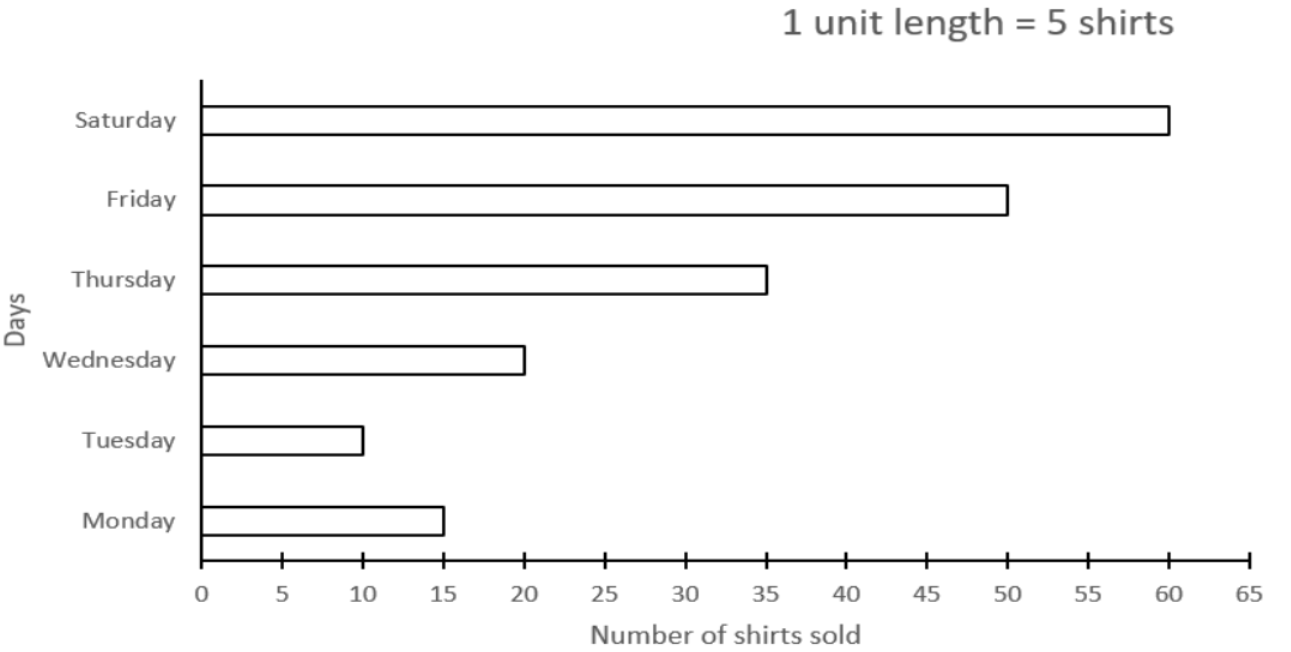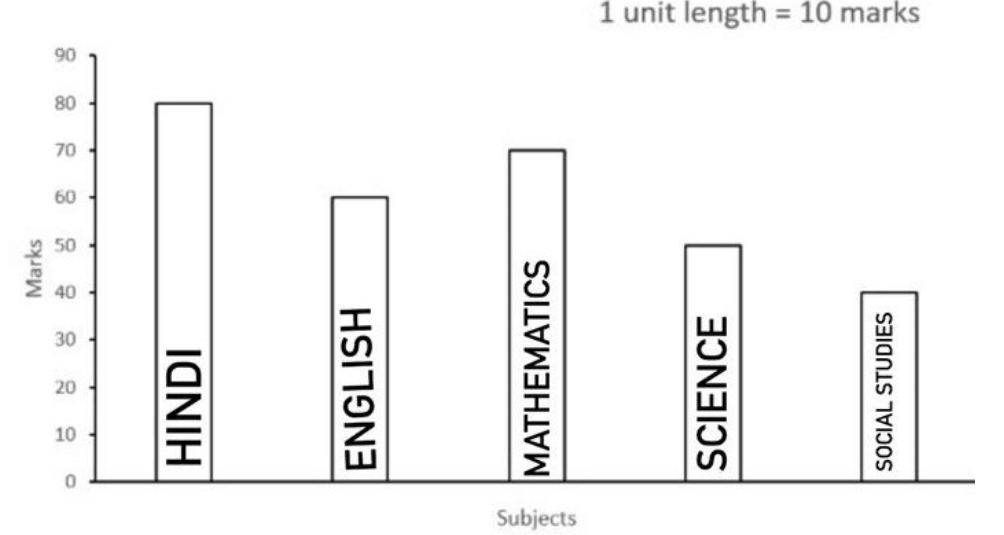Courses
Courses for Kids
Free study material
Free LIVE classes
More

# NCERT Solutions for Class 6 Maths Chapter 9 - ExerciseLIVE
Join Vedantu’s FREE Mastercalss

## NCERT Solutions for Class 6 Maths Chapter 9 Data Handling (Ex 9.3) Exercise 9.3

Free PDF download of NCERT Solutions for Class 6 Maths Chapter 9 Exercise 9.3 (Ex 9.3) and all chapter exercises at one place prepared by expert teacher as per NCERT (CBSE) books guidelines. Class 6 Maths Chapter 9 Data Handling Exercise 9.3 Questions with Solutions to help you to revise complete Syllabus and Score More marks. Register and get all exercise solutions in your emails.

Last updated date: 23rd Sep 2023
Total views: 506.7k
Views today: 6.06k

## Access NCERT Solutions for Class 6 Maths Chapter 9 – Data Handling

Exercise 9.3

Refer to page 12 for exercise 9.3 in the PDF.

1. The bar graph given below shows the amount of wheat purchased by the government during the year $1998 - 2002$.(a) In which year was the wheat production maximum?

Ans: Year with the highest bar is the year in which wheat production was maximum. In the given graph year $2002$ has the highest bar, therefore in $2002$, the wheat production was maximum.

(b) In which year was the wheat production minimum.

Ans: Year with the smallest bar is the year in which wheat production was minimum. In the given graph year $1998$ has the smallest bar, therefore in $1998$, the wheat production was minimum.

2. Observe this graph which shows the sale of shirts in a readymade shop from Monday to Saturday.(a) What information does the above graph give?

Ans: The given bar graph gives information about the number of shirts sold in a readymade shop from Monday to Saturday.

(b) What is the scale chosen on the horizontal line representing the number of shirts?

Ans: The scale chosen on the horizontal line representing number of shirts is

${\text{1 unit length}} = 5{\text{ shirts}}$.

(c) On which day were the maximum number of shirts sold? How many shirts were sold on that day?

Ans: Day with the highest bar is the day when sales of shirts were maximum. In the given graph Saturday has the highest bar, therefore on Saturday, a maximum number of shirts were sold. $60$ shirts were sold on Saturday.

(d) On which day were the minimum number of shirts sold?

Ans: Day with the smallest bar is the day when the sale of shirts was minimum. In the given graph Tuesday has the smallest bar, therefore on Tuesday, a minimum number of shirts were sold.

(e) How many shirts were sold on Thursday?

Ans: $35$ shirts were sold on Thursday.

3. Observe the bar graph which shows the marks obtained by Aziz in half-yearly examination in different subjects:(a) What information does the bar graph give?

Ans: The given bar graph gives information about the marks obtained by Aziz in half-yearly examinations in different subjects.

(b) Name the subject in which Aziz scored maximum marks.

Ans: The subject with the highest bar is the subject in which Aziz scored maximum marks. In the given bar graph Hindi is the highest bar, therefore in Hindi, Aziz scored the maximum marks.

(c) Name the subject in which Aziz scored minimum marks.

Ans: The subject with the smallest bar is the subject in which Aziz scored minimum marks. In the given bar graph Social Studies is the smallest bar, therefore in Social Studies, Aziz scored the minimum marks.

(d) State the name of the subjects and marks obtained in each of them.

Ans: Aziz scored $80$ marks in Hindi,$60$ in English, $70$ in Mathematics, $50$ in Science, $40$ in Social Studies.

## NCERT Solutions for Class 6 Maths Chapter 9 Data Handling Exercise 9.3

Opting for the NCERT solutions for Ex 9.3 Class 6 Maths is considered as the best option for the CBSE students when it comes to exam preparation. This chapter consists of many exercises. Out of which we have provided the Exercise 9.3 Class 6 Maths NCERT solutions on this page in PDF format. You can download this solution as per your convenience or you can study it directly from our website/ app online.

Vedantu in-house subject matter experts have solved the problems/ questions from the exercise with the utmost care and by following all the guidelines by CBSE. Class 6 students who are thorough with all the concepts from the Subject Maths textbook and quite well-versed with all the problems from the exercises given in it, then any student can easily score the highest possible marks in the final exam. With the help of this Class 6 Maths Chapter 9 Exercise 9.3 solutions, students can easily understand the pattern of questions that can be asked in the exam from this chapter and also learn the marks weightage of the chapter. So that they can prepare themselves accordingly for the final exam.

Besides these NCERT solutions for Class 6 Maths Chapter 9 Exercise 9.3, there are plenty of exercises in this chapter which contain innumerable questions as well. All these questions are solved/answered by our in-house subject experts as mentioned earlier. Hence all of these are bound to be of superior quality and anyone can refer to these during the time of exam preparation. In order to score the best possible marks in the class, it is really important to understand all the concepts of the textbooks and solve the problems from the exercises given next to it.

Do not delay any more. Download the NCERT solutions for Class 6 Maths Chapter 9 Exercise 9.3 from Vedantu website now for better exam preparation. If you have the Vedantu app in your phone, you can download the same through the app as well. The best part of these solutions is these can be accessed both online and offline as well.